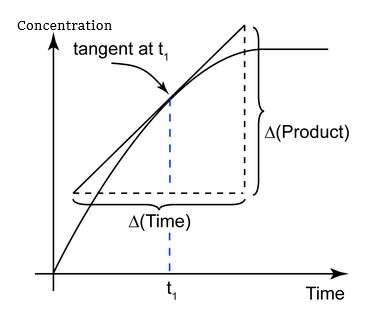# What Is Instantaneous Rate Of Reaction?

There are two ways to express the reaction rates and they are:

• Average rate of reaction
• Instantaneous rate of reaction

Instantaneous rate of reaction is defined as the change in concentration taking place at an infinitely small interval of time. It is usually expressed as either limit or as derivative.

The instantaneous reaction rate is given as:

 $$\begin{array}{l}\lim_{\Delta t\rightarrow 0}\frac{\Delta [concentration]}{\Delta t}\end{array}$$

There are two ways to determine the instantaneous reaction rate:

• By using data from experiments and finding the slope of the tangent from the concentration-time graph.
• By using data from experiments by getting their average rate and then determining the reaction rate from the slope of the tangents.

Consider a concentration-time graph for the reaction with P product.

The instantaneous rate of reaction is obtained from the slope of the tangent:(5)(0)